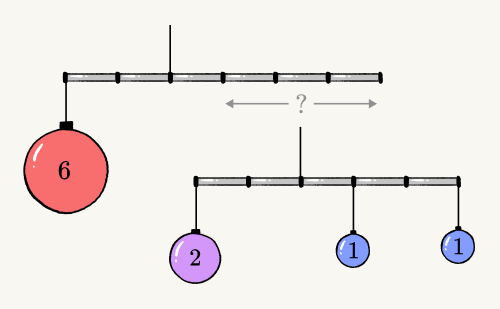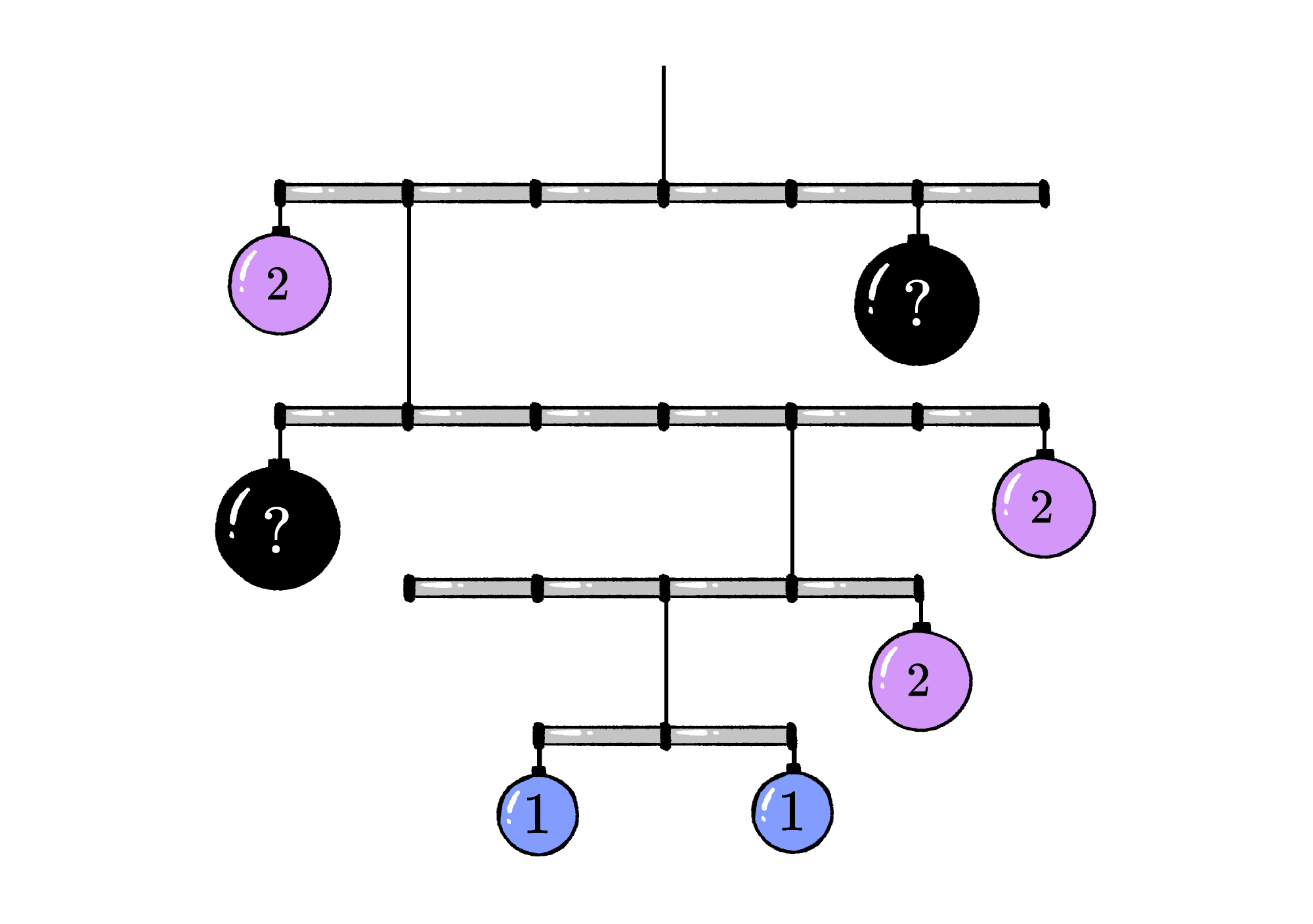Back

## Mysterious New Ornaments

Mobiles are dynamic sculptures that stay in perfect balance even as they twirl in the wind.

Balancing a mobile's decorative ornaments can be complicated. Mobiles often have multiple support rods that hang at different levels, adding layers of challenge. Can you add ornaments to the mobile below and keep it balanced?

A rod with hanging ornaments is perfectly balanced if it is supported at a particular point called its center of mass. This point is the average position of the masses hanging from the rod.

For example, suppose the ornament on the left is twice the weight of each of the other two ornaments. When the support is attached at the center of the rod, it tilts to the left. The red arrow shows the direction the rod begins to rotate. Where should you attach the string so that the rod is level and balanced?

The average position of the masses is calculated by summing each mass times its position, measured from a reference point that you choose, and then dividing by the total mass. If the reference point is the position of the larger mass, the rod has length $D,$ and the middle ornament is at position $\frac35 D,$ then the center of mass is located

\begin{aligned} \text{CM} &= \frac{2\times0+1\times \frac35 D+1\times D}{2+1+1}\\ &= \frac{2}{5}D \\ &=0.4 D\end{aligned}

to the right of the reference point (i.e. to the right of the left end of the rod).

To be level, each rod must be supported at the center of mass of the weight hanging from it. This includes ornaments and also hanging rods that make up the lower levels of the mobile.

For example, suppose you wanted to hang the rod we balanced earlier from another rod.Where should we attach the first rod to it so that it's level?

Assuming the rod itself is much lighter than the ornaments, we can ignore its weight, and the total weight of the hanging rod is $4$ times the weight of the smallest ornament. We can perform the same calculation to find the center of mass, with an unknown $x$ standing in for the position of the hanging rod.

This time, let's use as our reference point where the support is attached to the top rod. We want to find $x$ such that the center of mass is on this point. Each segment of the rod is length $L.$ Positions to the left will count as negative distances, and positions to the right will count as positive:

\begin{aligned} 0 &=\frac{6\times \left(-2L\right) + 4x}{6+4} \\ &=\frac{-12L +4x}{10} \\ \Rightarrow x&=3 L. \end{aligned}

This means when the rod is hung $3$ segments to the right of the support, the center of mass of the top level will be on our reference point, directly beneath the support.

# Today's Challenge

Someone rebalanced your mobile with two new ornaments. Some of the ornaments have known weights, which are shown, but the two black ornaments have unknown (and possibly different) weights.What's the weight of the heavier of the black ornaments?

×